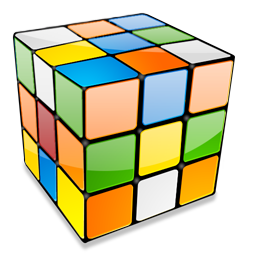#Combinatorics 2.0.0

.NET Standard 1.1 .NET Framework 4.6.1
`Install-Package Combinatorics -Version 2.0.0`
`dotnet add package Combinatorics --version 2.0.0`
`<PackageReference Include="Combinatorics" Version="2.0.0" />`
For projects that support PackageReference, copy this XML node into the project file to reference the package.
`paket add Combinatorics --version 2.0.0`
`#r "nuget: Combinatorics, 2.0.0"`
#r directive can be used in F# Interactive, C# scripting and .NET Interactive. Copy this into the interactive tool or source code of the script to reference the package.
```// Install Combinatorics as a Cake Addin

// Install Combinatorics as a Cake Tool
#tool nuget:?package=Combinatorics&version=2.0.0```

A combinatorics library providing combinations, permutations & variations. This nuget package wraps up Adrian Akison's original implementation. See: http://www.codeproject.com/Articles/26050/Permutations-Combinations-and-Variations-using-C-G

Product Versions
.NET net5.0 net5.0-windows net6.0 net6.0-android net6.0-ios net6.0-maccatalyst net6.0-macos net6.0-tvos net6.0-windows
.NET Core netcoreapp1.0 netcoreapp1.1 netcoreapp2.0 netcoreapp2.1 netcoreapp2.2 netcoreapp3.0 netcoreapp3.1
.NET Standard netstandard1.1 netstandard1.2 netstandard1.3 netstandard1.4 netstandard1.5 netstandard1.6 netstandard2.0 netstandard2.1
.NET Framework net45 net451 net452 net46 net461 net462 net463 net47 net471 net472 net48
MonoAndroid monoandroid
MonoMac monomac
MonoTouch monotouch
Tizen tizen30 tizen40 tizen60
Universal Windows Platform uap uap10.0
Windows Phone wpa81
Windows Store netcore netcore45 netcore451
Xamarin.iOS xamarinios
Xamarin.Mac xamarinmac
Xamarin.TVOS xamarintvos
Xamarin.WatchOS xamarinwatchos
Compatible target framework(s)
• #### .NETFramework 4.6.1

• No dependencies.

• #### .NETStandard 2.0

• No dependencies.

### NuGet packages (3)

Showing the top 3 NuGet packages that depend on Combinatorics:

IIR_Butterworth_CS_Library

C# code to calculate the coefficients of the Butterworth filter and to filter the data This code calculates the coefficients of the Band-pass, Band-stop, Low-pass and High-pass Butterworth filters. It also filters the data, but no zero-phase delay is applied. The namespace is IIR_Butterworth_CS_Library. Each filter function will return a 2 rows x N coefficients 2D vector, where Row 1 = Numerator and Row 2 = Denumerator. The method "Check_stability_iir" can be used to check the stability of the filter. Please, keep in mind that if the filter is unstable, numerical instability leading to numerical overflow might happen when the order selected is extremely high. If that situation occurs, the program might assign a default value of 10^10 at the denominator. Band-pass: the function is "double[][] Lp2bp(double W_f1, double W_f2, int order_filt)". The first two arguments are the two normalized cut-off frequencies (f1/NF, f2/NF), where NF is the Nyquist frequency. This means that the cutoff frequencies must be within the interval of (0,1). The last argument is the order. Please, keep in mind that if you enter order_filt = 2, the order of the order will be 2 * order_filt = 4; Band-stop: the function is "double[][] Lp2bs(double W_f1, double W_f2, int order_filt)". The first two arguments are the two normalized cut-off frequencies (f1/NF, f2/NF), where NF is the Nyquist frequency. This means that the cutoff frequencies must be within the interval of (0,1). The last argument is the order. Please, keep in mind that if you enter order_filt = 2, the order of the order will be 2 * order_filt = 4; Low-pass: the function is "double[][] Lp2hp(double W_f1, int order_filt)". The first argument is the normalized cut-off frequency (f/NF), where NF is the Nyquist frequency. This means that the cutoff frequency must be within the interval of (0,1). The last argument is the order; High-pass: the function is "double[][] Lp2lp(double W_f1, int order_filt)". The first argument is the normalized cut-off frequency (f/NF), where NF is the Nyquist frequency. This means that the cutoff frequency must be within the interval of (0,1). The last argument is the order; Check the stability of the filter: the method is "bool Check_stability_iir(double[][] coeff_filt)". The argument is the 2D array containing the filter coefficients. It returns "true" if the filter is stable, "false" if it is unstable. Filter the data: the method is "double[] Filter_Data(double[][] coeff_filt, double[] pre_filt_signal)". The two arguments are the filter coefficients and the signal to be filtered. It returns the filtered signal. This code has been written following the Matlab code, so the arguments of each function reflect the arguments that you should pass to the equivalent functions in Matlab. I tried to be consistent with the names of the functions, in case someone wants to compare this code with Matlab code. The only exception is the function Check_stability_iir, which is not present in Matlab. If you have any question and/or want to report bugs, please e-mail me (Ale) at: pressalex@hotmail.com

MNCD

Multi-layer Network Community Detection

Net.Math

Simple but handful math functions

### GitHub repositories (1)

Showing the top 1 popular GitHub repositories that depend on Combinatorics:

Repository Stars
Illumina/canvas
Canvas - Copy number variant (CNV) calling from DNA sequencing data